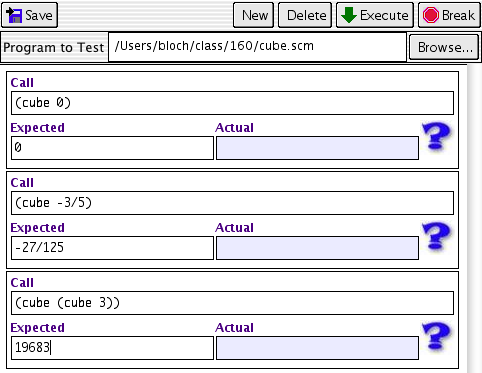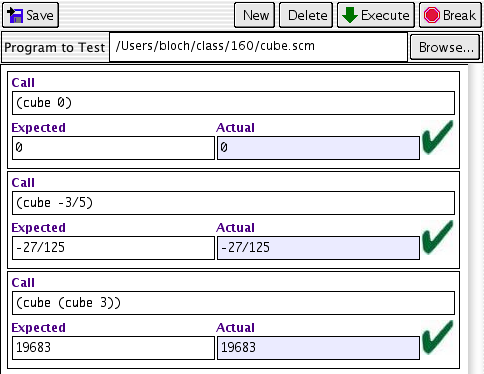# Examples

Write down one or more expressions that use this function (in correct Scheme syntax, so that if only the function were already defined, you could type them in and get answers), along with the "right answer" you expect each example to produce. This step is useful in at least three ways.
1. It allows you to try out the syntax people will use to invoke the function, and perhaps learn that your first guess at the syntax is inconvenient to use, so you should change the syntax before wasting a lot of time implementing it.
2. It allows you to discover, early on, that your contract wasn't quite right, and that you actually want different kinds of information going in or out. If that happens, change the contract now and re-write your examples accordingly; that will waste less time than going on and having to change the contract later.
3. It gives you a ready source of test cases, already in legal Scheme syntax, thus removing one possible excuse for skipping the Testing step, below.

Start with the simplest possible examples, then work up to more and more complicated examples. One way to write this is in a Scheme comment, e.g.

```; Cube function: find the third power of a number
; cube: number => number
; (cube 0) => 0
; (cube -3/5) => -27/125
; (cube (cube 3)) => 19683
```

Another is to write it directly in the Definitions window, specifying the correct answers with "should be..." , but then insert the function definition in front of it, e.g.

```; Cube function: find the third power of a number
; cube: number => number
function definition will go here
(cube 0) "should be 0"
(cube -3/5) "should be -27/125"
(cube (cube 3)) "should be 19683"
```As of DrScheme version 203, there's a third way: you can create a test suite with each of your test cases and its correct answers. Initially, the suite will look like this:After you've written the function definition and saved it, you can press the "Execute" button on the test suite and the result should look like this: